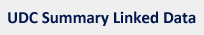Notation
5
Caption
MATHEMATICS. NATURAL SCIENCES
Scope Note
Class here generalities about the pure sciences and the exact or mathematical sciences (including astronomy, mechanics and mathematical physics)
Examples
 5(091) History of natural sciences